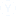If 137 + 276 = 435 how much is 731 + 672 ?
If 137 + 276 = 435 how much is 731 + 672 ? (A) 534 (B) 1403 (C) 1623 (D) 15311-7 of  7
• B

• (C) 1623

• 1623

• Option C is the answer. Since the given sum is added by 20 in the question. In the same way 220 is added to the sum in the next question..

• not clear

• A

• First look at the ones digit. Something that ends in 7 plus something that ends in 6 usually ends in 3.  But in this case the answer ends in 5,  so we must not be working in base 10!  Instead, 6+7=13 must be 5 plus a multiple of our base. So either it's base 8, base 4, or base 2.  But it can't be base 4 or base 2, since some of the digits are bigger than 4. The only possibility left is base 8.

So is it really base 8?  Let's check:

6+7 = 13 (base 10) which is 15 (base 8).  So write down a 5 and carry the one.
3+7+1 = 11 (base 10), which is 13 (base 8). Write down a 3 and carry the one.
1+2+1=4.

So 137 (base 8) + 276 (base 8) = 435 (base 8). So far so good!

Now do the other addition base 8:

1+2=3, so the last digit is 3
3+7=10 (base 10) = 12 (base 8), so the next digit is 2.  Carry the 1.
7+6+1 = 14 (base 10) = 16 (base 8).Other Related DiscussionsPractice Mock Test
Aptitude